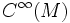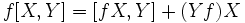# Corollary of Leibniz rule for Lie bracket

(diff) ← Older revision | Latest revision (diff) | Newer revision → (diff)
This is an identity that uses the Leibniz rule to measure the failure of the Lie bracket operation from being$C^\infty$-linear.
Let$X,Y$ be smooth vector fields on a differential manifold$M$ and$f$ be in$C^\infty(M)$. We then have:$f[X,Y] = [fX,Y] + (Yf)X$